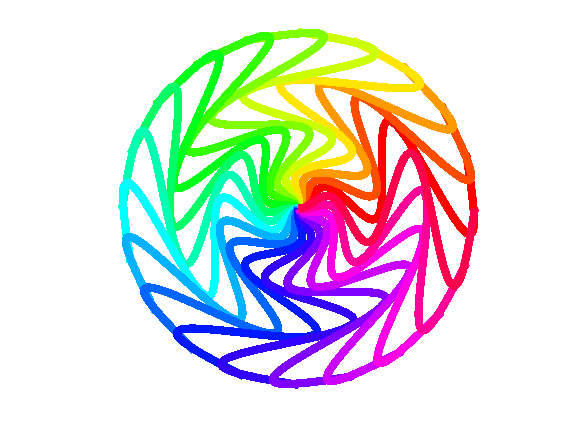# kaleidoscopesChen Lin on 4 Oct 2021
• 26
• 456
• 15
• 0
• 245
% This is a demo created by MathWorks community team.
% Author: Ned Gulley
% Please do not vote on this demo entry.
x = linspace(0, 4*pi);
y = 0.5*x.*sin(x);
h1 = plot(x, y, LineWidth=6);
k = 20;
c = hsv(k);
for n = 1:k
h2 = copyobj(h1, gca);
t = hgtransform;
set(h2,Parent=t,Color=c(n,:))
theta = n*2*pi/k;
rot = makehgtform(ZRotate=theta);
set(t, Matrix=rot);
end
axis equal
axis off